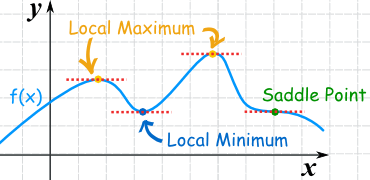# Derivatives made easy 3a) Maximum and minimum explained

Hi,
in school we learn how to derive right now. The teacher does not do very good job. The class does not know about limits and they only learned power rule and no other rule for derivation though at the same time they learn about maxima and minima.

When you derive function you can find lot of useful informations about it. Today I will talk about minima and maxima. Those are points where the function turns around creates “hill” or “valley” – maxima or minima.Some fictional function.

In the function above (f-blue line) you can see couple of points marked. Two orange points are maxima, blue is minima and green is special to which I will get in another post.

As I have already said quite few times, when you derive function you will find out what is the slope (how much up or down) at one point. If it does not go up or down it is zero, those are the maxima and minima you are looking for. Right before maximum you have positive slope, the function goes up towards maximum. Right after maximum you have negative slope. What is between if the function is smooth? Zero. When we derive function, lets say function like this:

f(x)=2x²    We get (according to power rule, if you do not know it ignore it right now):

f'(x)=4x    The apostrophe means that it has been derived.

Now when does this derivation equals zero? In other words? When is the slope zero?

Only when x=0 because f'(0)=4*0 = 0 

The original function has minimum or maximum on the point when x=0 . Tricky is that you do not know if it is minimum or maximum which needs some more work and I will get to it in another post.

Dragallur

I will also talk about power rule, multiplication rule and so on in the future.

To get the coordinates of the maximum or minimum you just plug the solution of the derivative to original function and you will get the y coordinate.

If you work with higher order polynomials like x³ you might easily get more solutions which means more minimas and maximas.

If you plot the derivation you will get the slope anywhere.

PS: sometimes I see minima or minimum and maxima or maximum so I use both as I want.